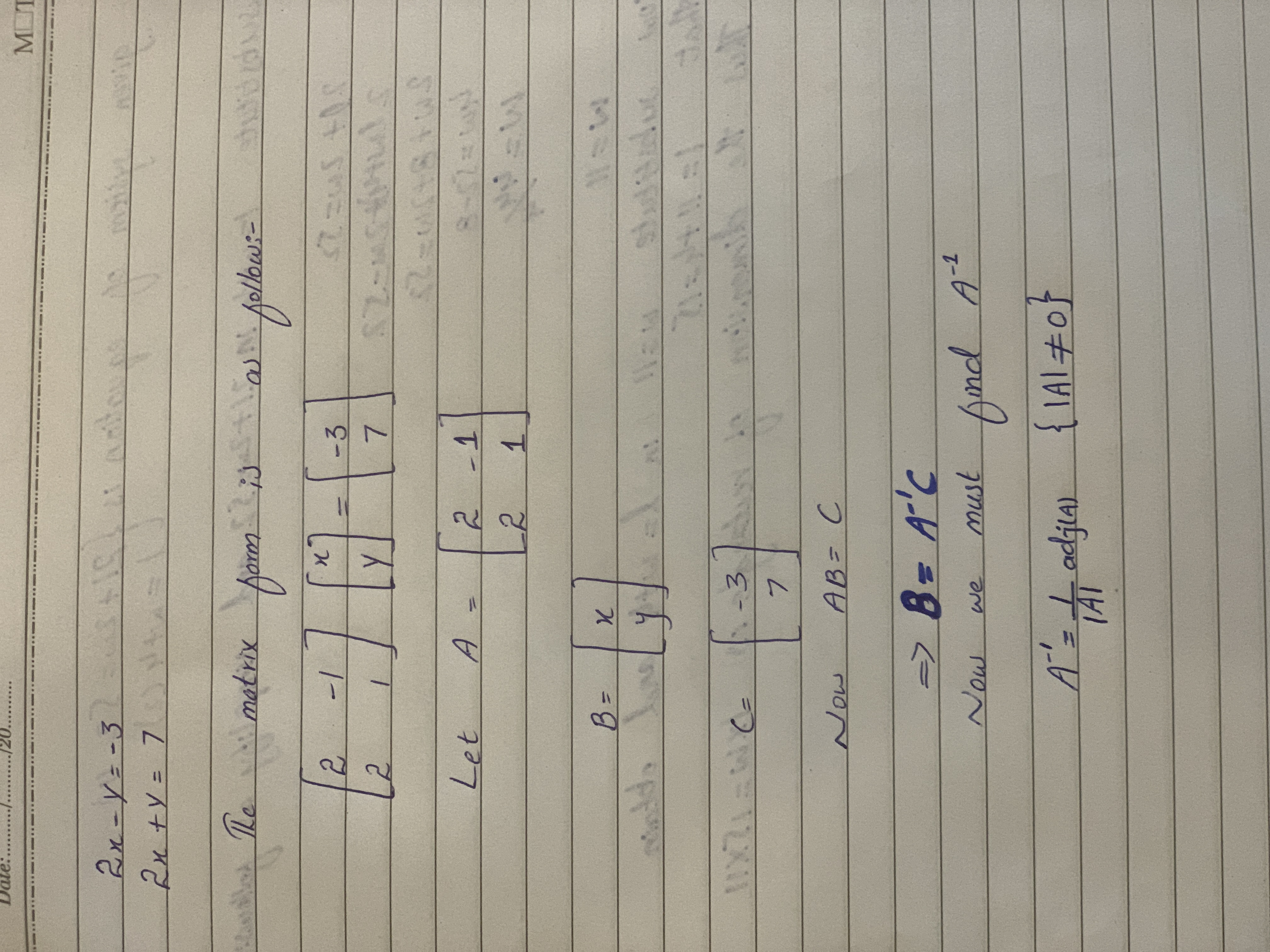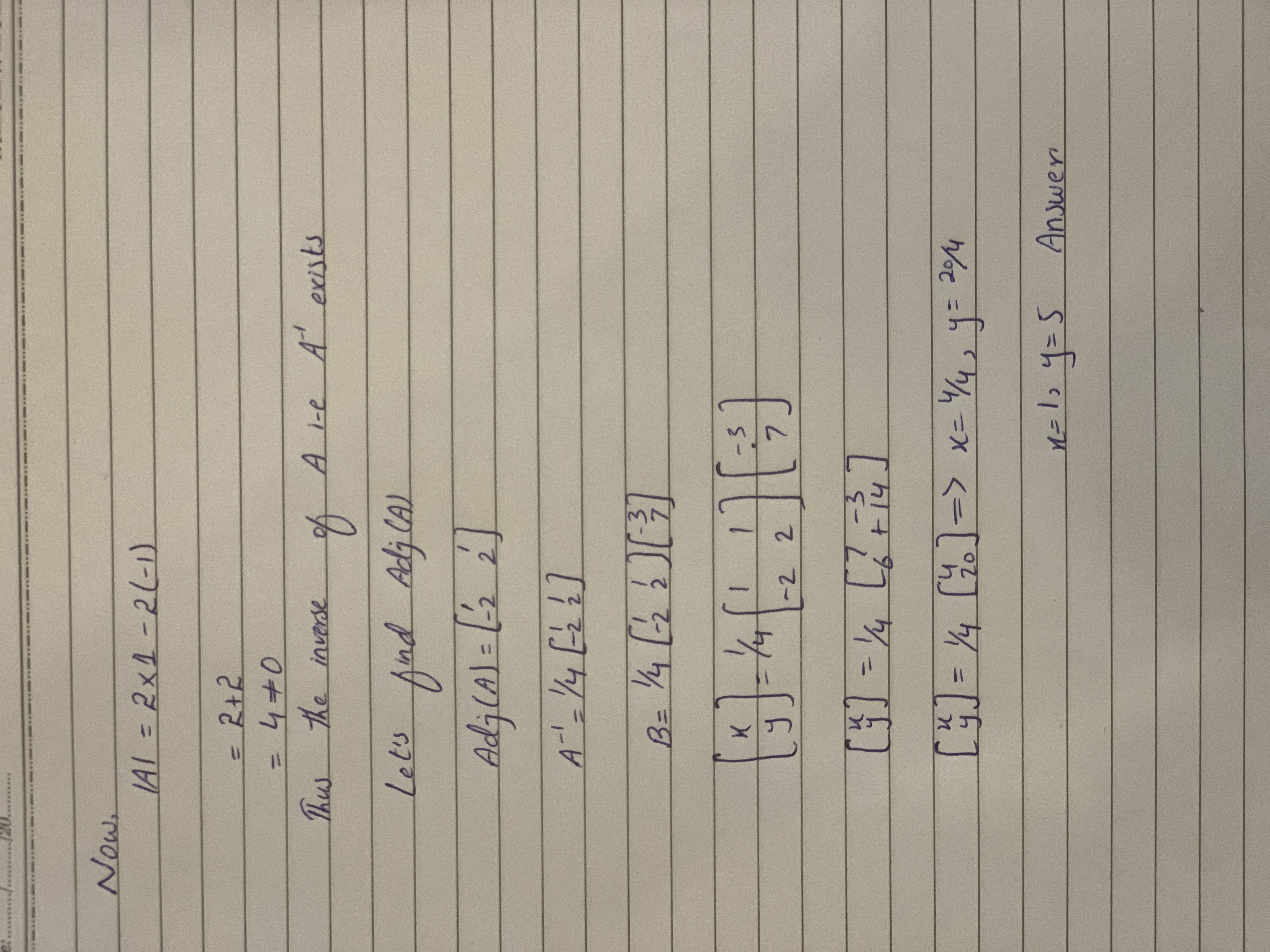Ask question

# Use an inverse matrix to solve system of linear equations. (a) 2x-y=-3 2x+y=7 (b) 2x-y=-1 2x+y=-3# Use an inverse matrix to solve system of linear equations. (a) 2x-y=-3 2x+y=7 (b) 2x-y=-1 2x+y=-3

Question
Forms of linear equationsasked 2020-11-12
Use an inverse matrix to solve system of linear equations.
(a)
2x-y=-3
2x+y=7
(b)
2x-y=-1
2x+y=-3

## Answers (1)2020-11-13### Relevant Questionsasked 2021-02-18

Use an inverse matrix to solve system of linear equations.
$$x+2y=-1$$
$$x-2y=3$$asked 2020-11-06
A small grocer finds that the monthly sales y (in $) can be approximated as a function of the amount spent advertising on the radio $$x_1$$ (in$) and the amount spent advertising in the newspaper $$x_2$$ (in $) according to $$y=ax_1+bx_2+c$$ The table gives the amounts spent in advertising and the corresponding monthly sales for 3 months. $$\begin{array}{|c|c|c|}\hline \text { Advertising, } x_{1} & \text { Advertising, } x_{2} &\text{sales, y} \\ \hline 2400 & { 800} & { 36,000} \\ \hline 2000 & { 500} & { 30,000} \\ \hline 3000 & { 1000} & { 44,000} \\ \hline\end{array}$$ a) Use the data to write a system of linear equations to solve for a, b, and c. b) Use a graphing utility to find the reduced row-echelon form of the augmented matrix. c) Write the model $$y=ax_1+bx_2+c$$ d) Predict the monthly sales if the grocer spends$250 advertising on the radio and $500 advertising in the newspaper for a given month.asked 2021-03-12 Determine whether the ordered pair is a solution to the given system of linear equations. (5,3) $$x-y=2$$ $$x+y=8$$asked 2021-02-19 For the given a system of linear equations 4x+y-5z=8 -2x+3y+z=12 3x-y+4z=5 Use matrix inversion to solve simultaneous equations.asked 2020-10-19 The purchase price of a home y (in$1000) can be approximated based on the annual income of the buyer $$x_1$$ (in $1000) and on the square footage of the home $$x_2 (\text{ in } 100ft^2)$$ according to $$y=ax_1+bx_2+c$$ The table gives the incomes of three buyers, the square footages of the home purchased, and the corresponding purchase prices of the home. a) Use the data to write a system of linear equations to solve for a, b, and c. b) Use a graphing utility to find the reduced row-echelon form of the augmented matrix. c) Write the model $$y=ax_1+bx_2+c$$ d) Predict the purchase price for a buyer who makes$100000 per year and wants a $$2500ft^2$$ home.asked 2021-03-10

A system of linear equations is given below.
$$2x+4y=10$$
$$\displaystyle-{\frac{{{1}}}{{{2}}}}{x}+{3}={y}$$
Find the solution to the system of equations.
A. (0, -3)
B. (-6, 0)
C. There are infinite solutions.
D. There are no solutions.asked 2021-03-15
Use back-substitution to solve the system of linear equations.
$$\displaystyle{b}{e}{g}\in{\left\lbrace{c}{a}{s}{e}{s}\right\rbrace}{x}&-{y}&+{5}{z}&={26}\backslash&\ \ \ {y}&+{2}{z}&={1}\backslash&&\ \ \ \ \ {z}&={6}{e}{n}{d}{\left\lbrace{c}{a}{s}{e}{s}\right\rbrace}$$
(x,y,z)=()asked 2020-10-23

Determine if (1,3) is a solution to the given system of linear equations.

$$5x+y=8$$

$$x+2y=5$$asked 2021-03-05

Find the augmented matrix for the following system of linear equations:
$$3x+7y-20z=-4$$
$$5x+12y-34z=-7$$asked 2021-03-10
Use Cramer's rule to solve the given system of linear equations.
$$\displaystyle{x}_{{{1}}}-{x}_{{{2}}}+{4}{x}_{{{3}}}=-{2}$$
$$\displaystyle-{8}{x}_{{{1}}}+{3}{x}_{{{2}}}+{x}_{{{3}}}={0}$$
$$\displaystyle{2}{x}_{{{1}}}-{x}_{{{2}}}+{x}_{{{3}}}={6}$$
...